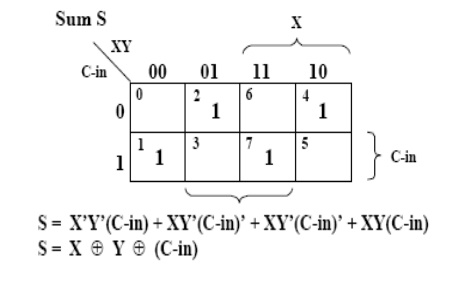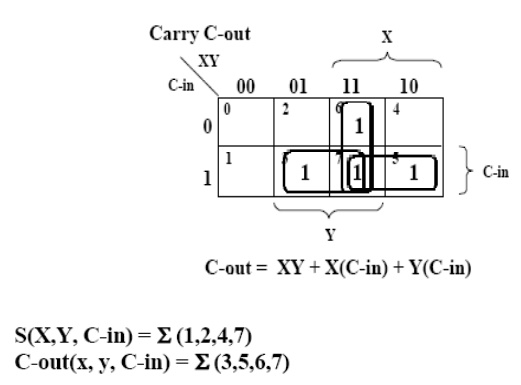## Chapter: Digital Electronics : Combinational Circuits

Design a logic circuit to add two bits and produce a sum bit and a carry bit. Two inputs and two outputs are needed. Let us call the inputs x and y, and the outputs S and C.

Design a logic circuit to add two bits and produce a sum bit and a carry bit. Two inputs and two outputs are needed. Let us call the inputs x and y, and the outputs S and C.–   Gives “carry out” and “sum”

–   1 AND and 1 XOR gate

Adding two single-bit binary values, X, Y with a carry input bit C-in produces a sum bit S and a carry out C-out bit.–   For adding 2 bits plus a “carry in”

–   Gives “carry out” and “sum”

–   2 ANDs, 2 XORs, and 1 OR

## FULL ADDER CIRCUIT USING AND-OR:## FULL ADDER CIRCUIT USING XOR:Study Material, Lecturing Notes, Assignment, Reference, Wiki description explanation, brief detail
Digital Electronics : Combinational Circuits : Half Adder and Full Adder |